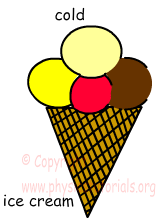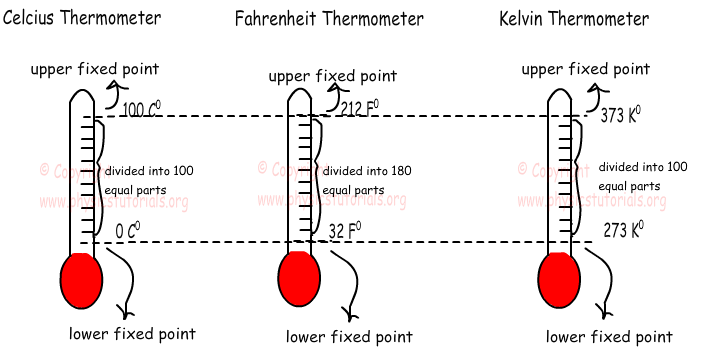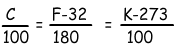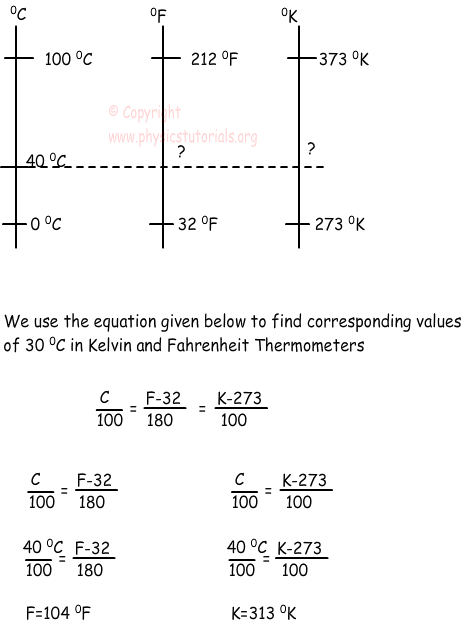## Temperature

TemperatureAll matters are formed from atoms and molecules. In microscopic view we see that all particles in a matter are in random motion, they are vibrating, colliding randomly. We learned in previous sections that particle has kinetic energy if it moves. Thus, in an object all particles have kinetic energies because of their random motions. Temperature is the quantity which is directly proportional to the average kinetic energy of the atoms of matter. Be careful, it is not energy, it just show the quantity of average kinetic energy of one atom or one molecule.In daily life we use some terms like hot, cold or warm. All these terms are used with respect to another reference matter. For example, you say that a glass of boiling water is hotter than the ice cream. Be careful, ice cream is our reference matter.

We measure temperature of matters with a device called thermometer. There are three types of thermometer, Celsius Thermometer, Fahrenheit Thermometer and Kelvin Thermometer. Look at the given picture to see how we scale thermometers.In Celsius thermometer, lower fixed point is 0 ºC and upper fixed point is 100 ºC, in Fahrenheit thermometer lower fixed point is determined as 32 ºF and upper fixed point as 212 ºF and finally, lower fixed point of Kelvin thermometer is 273 ºK and upper fixed point is 373 ºK. These temperatures are determined with considering the freezing point and boiling point of water.

We can convert the measurements of Celsius to Kelvin or Fahrenheit to Kelvin, Celsius by using following equations.Example: Find the values of 30 ºC in Fahrenheit and Kelvin thermometers.Heat Temperature and Thermal Expansion Exams and Problem Solutions

Author: